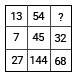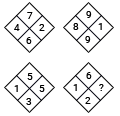# Finding the missing character: Concepts & tricks

Introduction
Questions, which ask to insert the missing number, come under this category.  In most cases one of the following will be given.
1. A diagram or a set of diagrams2. Or a matrix3. Or  any random figure e.g. star
Each of these has some characters. These characters can be numbers or letters or a combination of number and letters that follow a particular pattern. We need to crack the reasoning or logic behind this pattern so as to find the missing term.
Most common patterns
Different types of patterns could be there, some of the patterns are as following
1. It could be sum of two numbers divided by a constant
2. It could be average of numbers
3. It could be in the form of alphabets, where alphabets are increased by constant or increased by square of numbers or increased by prime numbers
4. It could be the difference of product of two diametrically opposite numbers.
5. It could be the difference of sum of adjacent numbers.
6. The difference of the numbers could be in the pattern   13±1,23±1,  and so on.
7. The difference of the numbers could be in the pattern 12±1, 22±1, and so on.
8. Numbers could be 12,22,32and so on  or 13,23, 33… and so on.
9. The difference could be prime numbers or difference could be square of prime numbers.
10. The difference could be in the form of N2±N or N3±N.
11. The difference could be in the form of ×N+N or ×2+1,×2+2,×2+3…and so on.
12. The difference could be in the form of×2±1 alternatively.
13. Numbers could be ×1,×2,×3…… and so on.